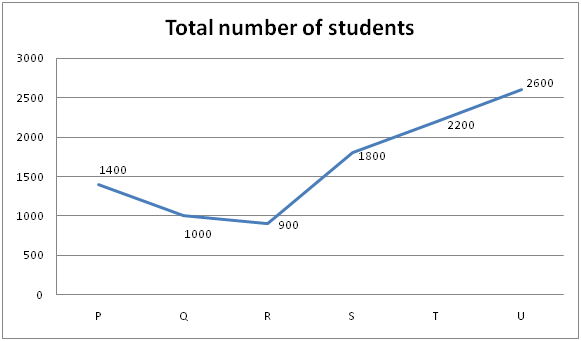# NIACL AO Mains– Quantitative Aptitude Questions Day- 08

Dear Readers, Bank Exam Race for the Year 2019 is already started, To enrich your preparation here we have providing new series of Practice Questions on Quantitative Aptitude – Section. Candidates those who are preparing for NIACL AO Mains 2019 Exams can practice these questions daily and make your preparation effective.

[WpProQuiz 5126]

Directions (Q. 1 – 5): What approximate value should come in place of (?) in the following questions?

1) (21/47) ÷ (179/234) × 141 + 3278.12 – 42 % of 899 =?

a) 2982

b) 3156

c) 2844

d) 2353

e) 1890

2) (3/17.92) × 431 + (3.72/14) × 209 + 70% of 499 =? – 1335

a) 2356

b) 2192

c) 1817

d) 1624

e) 2530

3) 90% of 12599 + 120% of 32999 – 635.92 =?

a) 42728

b) 50304

c) 33627

d) 39756

e) 45130

4) (3/11) of 186.58 × 25.12 ÷ 5 – ? = 65.31 ÷ 5 + 24.98

a) 234

b) 292

c) 305

d) 217

e) 350

5) 12 (¾) – 5 (7/9) + 11 (1/5) – 8 (1/9) =? – 3 (½)

a) 14

b) 25

c) 8

d) 33

e) 42

Directions (Q. 6 – 10): Study the following information carefully and answer the given questions.

The following line graph shows the total number of students from 6 different colleges in a certain year.6) 23 % and 32 % of the students from college P and R respectively are going to symposium in college A to participate all the events. 52 % of the students from college A doesn’t participate any event, which is equal to 78 % of average number of students from all the given colleges together (i.e., college P, Q, R, S, T and U). Find the total number of students participated in symposium events from college P, R and A together?

a) 1798

b) 1852

c) 1644

d) 1936

e) None of these

7) Total number of students from college P and R together is what percentage of total number of students from college Q and T together?

a) 85.275 %

b) 66.525 %

c) 52.125 %

d) 71.875 %

e) None of these

8) If the ratio between the total number of girls to that boys from college S and college T is 43 : 32 and 27 : 23 respectively, then find the difference between the total number of  girls to that of boys in college S and T together?

a) 320

b) 440

c) 560

d) 680

e) None of these

9) Find the total number of students in college B, if the total number of students in college B is 380 less the average number of students from all the given colleges together (i.e., College P, Q, R, S, T and U)?

a) 1350

b) 1480

c) 1270

d) 1560

e) None of these

10) Total number of students from college P, R and U together is approximately what percentage more/less than the total number of students from college Q, S and T together?

a) 2 % less

b) 15 % more

c) 15 % less

d) 2 % more

e) 23 % less

Direction (1-5) :

(21/47) ÷ (180/235) × 140 + 3278 – 42 % of 900 = x

(21/47)*(235/180)*140 + 3278 – (42/100)*900 = x

X = 82 + 3278 – 378 = 2982

(3/18) × 432 + (4/14) × 210 + (70/100)*500 = x – 1335

72 + 60 + 350 + 1335 = x

X = 1817

90% of 12599 + 120% of 32999 – 635.92 = x

X = (90/100)*12600 + (120/100)*33000 – 636

X = 11340 + 39600 – 636

X = 50304

(3/11)*187 × 25 ÷ 5 – x = (65/5) + 25

255 – 13 – 25 = x

X = 217

12 (¾) – 5 (7/9) + 11 (1/5) – 8 (1/9) + 3 (½) = x

X = 13 – 6 + 11 – 8 + 4

X = 14

Direction (6-10) :

Total number of students from college A

= > (78/100)*[(1400 + 1000 + 900 + 1800 + 2200 + 2600)/6]*(100/52)

= > (78/100)*(9900/6)*(100/52)

= > 2475

The total number of students participated in symposium events from college P, R and A together

= > 1400*(23/100) + 900*(32/100) + 2475*(48/100)

= > 322 + 288 + 1188

= > 1798

Total number of students from college P and R together

= > 1400 + 900 = 2300

Total number of students from college Q and T together

= > 1000 + 2200 = 3200

Required % = (2300/3200)*100 = 71.875 %

The total number of girls in college S and T together

= > 1800*(43/75) + 2200*(27/50)

= > 1032 + 1188 = 2220

The total number of boys in college S and T together

= > 1800*(32/75) + 2200*(23/50)

= > 768 + 1012 = 1780

Required difference = 2220 – 1780 = 440

The total number of students in college B

= > [(1400 + 1000 + 900 + 1800 + 2200 + 2600)/6] – 380

= > (9900/6) – 380

= > 1270

Total number of students from college P, R and U together

= > 1400 + 900 + 2600 = 4900

Total number of students from college Q, S and T together

= > 1000 + 1800 + 2200 = 5000

Required % = [(5000 – 4900)/5000]*100 = 2 % less